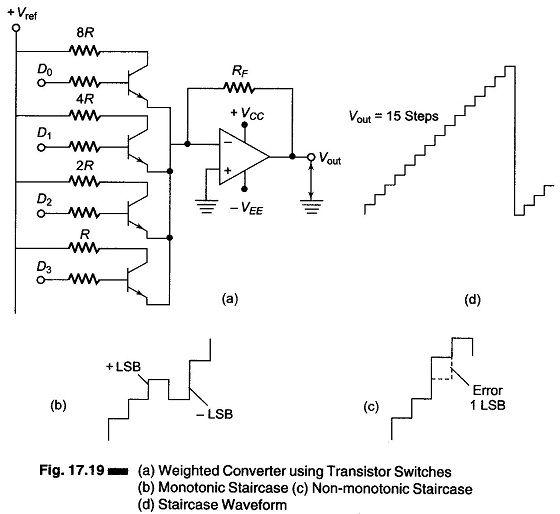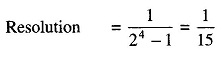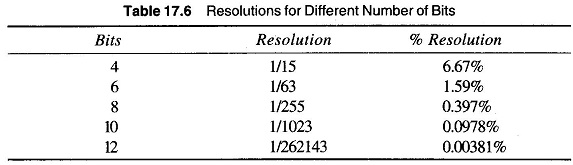## Weighted Converter Using Transistor Switches (Current Switch):

Figure 17.19 (a) shows a circuit diagram of a weighted converter, using transistor switches.When the D bit is high (logic 1), it produces enough base current to saturate the transistor. When the D bit is low (logic 0), the base current is zero and the transistor remains off. Therefore, each transistor is in either saturation or cutoff, and acts as an open circuit or closed switch. If the D bits are the output of a counter, the output is 0000, 0001, …, 1111. The digital signal is converted into a continuous signal i.e. D/A conversion has taken place. One step is equal to an LSB increment. The resolution of the staircase is defined as the ratio of the LSB increment to the maximum output. ThereforeThis is sometimes given as 1 part in 15 and

% Resolution = Resolution x 100

For a 4 bit converter, the % resolution is 1/15 x 100 = 6.67%.

The number of bits gives the resolution and an estimates number of steps. This converter is a weighted resistor converter. The accuracy of the output depends mainly on the tolerances of the weighted resistors. If they are exactly R, 2R, 4R and 8R, then all steps will be equal. If the resistances do not have these tolerances, the stairs (steps) will have non-equal steps. The various % resolutions for different numbers of bits are given in Table 17.6. The output staircase waveform is shown in Fig. 17.19(d).When the weighted resistances used are of poor quality, the staircase produced will not be monotonic.

### Monotonicity

A monotonic D/A converter is one that produce an increment in output for each increment in the input. The staircases voltages are nonmonotonic shown in Fig. 17.19 (c). For a monotonic converter the error permissible is less than + 1/2 LSB. Considering the worst case of + 1/2 LSB error, if it is followed by a — 1/2 LSB error, a critical level is produced, where the monotonicity is not lost as shown in Fig. 17.19 (b).